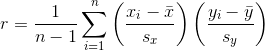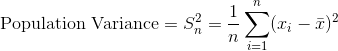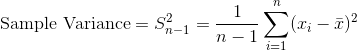# 二十一、统计学

## 贝塞尔校正## 演示中心极限定律

``````# 导入包
import pandas as pd
import numpy as np

# 将 matplotlib 设为内联
%matplotlib inline

# 创建空的数据帧
population = pd.DataFrame()

# 创建一列，它是来自均匀分布的 10000 个随机数
population['numbers'] = np.random.uniform(0,10000,size=10000)

# 绘制得分数据的直方图
# 这确认了数据不是正态分布的
population['numbers'].hist(bins=100)

# <matplotlib.axes._subplots.AxesSubplot at 0x112c72710>
````````````# 查看数值的均值
population['numbers'].mean()

# 4983.824612472138

# 创建列表
sampled_means = []

# 执行 1000 次
for i in range(0,1000):
# 从总体中随机抽取 100 行
# 计算它们的均值，附加到 sampled_means
sampled_means.append(population.sample(n=100).mean().values)

# 绘制 sampled_means 的直方图
# 它很明显是正态分布的，中心约为 5000
pd.Series(sampled_means).hist(bins=100)

# <matplotlib.axes._subplots.AxesSubplot at 0x11516e668>
````````````# 查看 sampled_means 的均值
pd.Series(sampled_means).mean()

# 4981.465310909289

# 将样本均值的均值减去真实的总体均值
error = population['numbers'].mean() - pd.Series(sampled_means).mean()

# 打印
print('The Mean Sample Mean is only %f different the True Population mean!' % error)

# The Mean Sample Mean is only 2.359302 different the True Population mean!
``````

## 皮尔逊相关系数

``````import statistics as stats

x = [1,2,3,4,5,6,7,8,9]
y = [2,1,2,4.5,7,6.5,6,9,9.5]
````````````# 创建函数
def pearson(x,y):

# 创建 n，数据中的观测数量
n = len(x)

# 创建列表来储存标准得分
standard_score_x = []
standard_score_y = []

# 计算 x 的均值
mean_x = stats.mean(x)

# 计算 x 的标准差
standard_deviation_x = stats.stdev(x)

# 计算 y 的均值
mean_y = stats.mean(y)

# 计算 y 的标准差
standard_deviation_y = stats.stdev(y)

# 对于 x 中的每个观测
for observation in x:

# 计算 x 的标准得分
standard_score_x.append((observation - mean_x)/standard_deviation_x)

# 对于 y 中的每个观测
for observation in y:

# 计算 y 的标准得分
standard_score_y.append((observation - mean_y)/standard_deviation_y)

# 将标准得分加在一起，求和，然后除以 n-1，返回该值
return (sum([i*j for i,j in zip(standard_score_x, standard_score_y)]))/(n-1)

# 展示皮尔逊相关系数
pearson(x,y)

# 0.9412443251336238
``````

## 概率质量函数（PMF）

``````# 加载库
import matplotlib.pyplot as plt

# 创建一些随机整数
data = [3,2,3,4,2,3,5,2,2,3,3,5,2,2,5,6,2,2,2,3,6,6,2,4,3,2,3]

# 创建字典来储存计数
count = {}

# 对于数据中的每个值
for observation in data:
# 键为观测，值递增
count[observation] = count.get(observation, 0) + 1

# 计算观测数量 observations
n = len(data)

# 创建字典
probability_mass_function = {}

# 对于每个唯一值
for unique_value, count in count.items():
# 将计数归一化，通过除以数据量，添加到 PMC 字典
probability_mass_function[unique_value] = count / n

# 绘制概率质量函数
plt.bar(list(probability_mass_function.keys()), probability_mass_function.values(), color='g')
plt.show()
``````## Spearman 排名相关度

``````import numpy as np
import pandas as pd
import scipy.stats

# 创建两列随机变量
x = [1,2,3,4,5,6,7,8,9]
y = [2,1,2,4.5,7,6.5,6,9,9.5]
``````

Spearman 的排名相关度，是变量的排名版本的皮尔逊相关系数。

``````# 创建接受 x 和 y 的函数
def spearmans_rank_correlation(xs, ys):

# 计算 x 的排名
#（也就是排序后元素的位置）
xranks = pd.Series(xs).rank()

# 计算 y 的排名
yranks = pd.Series(ys).rank()

# 在数据的排名版本上，计算皮尔逊相关系数
return scipy.stats.pearsonr(xranks, yranks)

# 运行函数
spearmans_rank_correlation(x, y)

# 0.90377360145618091

# 仅仅检查我们的结果，使用 Scipy 的 Spearman
scipy.stats.spearmanr(x, y)

# 0.90377360145618102
``````

## T 检验

``````from scipy import stats
import numpy as np

# 创建 20 个观测的列表，从均值为 1，
# 标准差为 1.5 的正态分布中随机抽取
x = np.random.normal(1, 1.5, 20)

# 创建 20 个观测的列表，从均值为 0，
# 标准差为 1.5 的正态分布中随机抽取
y = np.random.normal(0, 1.5, 20)
``````

### 单样本双边 T 检验

``````# 运行 T 检验来检验 x 的均值和 0 相比，是否有统计学显著的差异
pvalue = stats.ttest_1samp(x, 0)

# 查看 p 值
pvalue

# 0.00010976647757800537
``````

### 双样本非配对等方差双边 T 检验

``````stats.ttest_ind(x, y)

# 0.00035082056802728071

stats.ttest_ind(x, y, equal_var=False)

# 0.00035089238660076095
``````

### 双样本配对双边 T 检验

``````stats.ttest_rel(x, y)

# 0.00034222792790150386
``````

## 方差和标准差

``````# 导入包
import math

# 创建值的列表
data = [3,2,3,4,2,3,5,2,2,33,3,5,2,2,5,6,62,2,2,3,6,6,2,23,3,2,3]
````````````# 计算 n
n = len(data)

# 计算均值
mean = sum(data)/len(data)

# 从均值创建所有观测的差
all_deviations_from_mean_squared = []

# 对于数据中的每个观测
for observation in data:

# 计算到均值的差
deviation_from_mean = (observation - mean)

# 计算平方
deviation_from_mean_squared = deviation_from_mean**2

# 将结果添加到列表
all_deviations_from_mean_squared.append(deviation_from_mean_squared)

# 对于列表中所有平方差求和
sum_of_deviations_from_mean_squared = sum(all_deviations_from_mean_squared)

# 除以 n
population_variance = sum_of_deviations_from_mean_squared/n

# 展示方差
population_variance

# 160.78463648834017
``````

``````# 计算总体方差的平方根
population_standard_deviation = math.sqrt(population_variance)

# 打印总体标准差
population_standard_deviation

# 12.68008818929664
``````